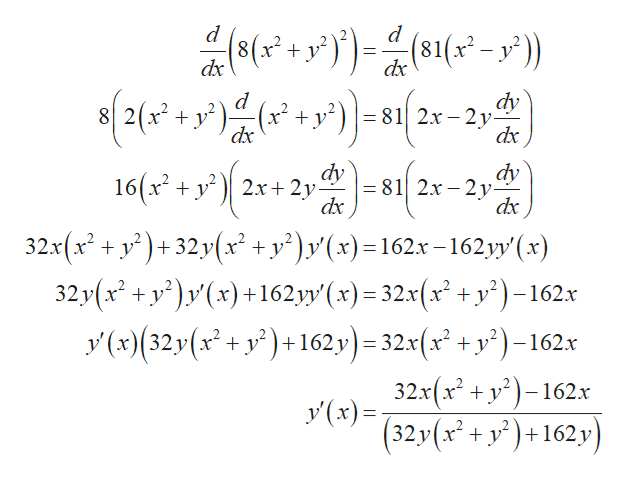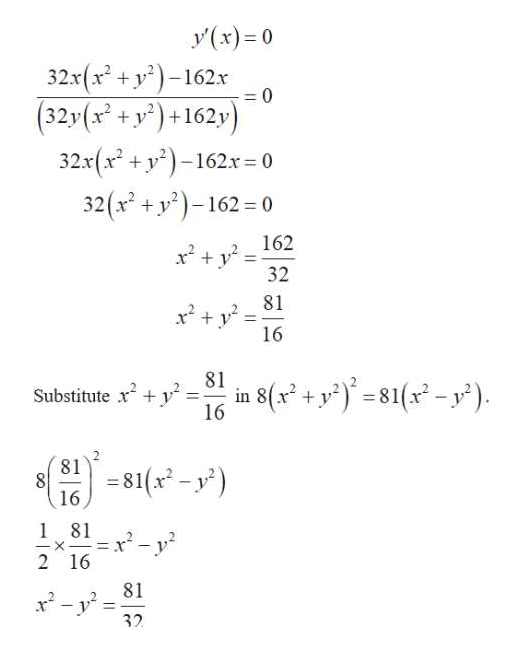# Find the points on the lemniscate where the tangent is horizontal.8(x2 + y2)2 = 81(x2 − y2)

Question
387 views

Find the points on the lemniscate where the tangent is horizontal.

8(x2 + y2)2 = 81(x2 − y2)

check_circle

Step 1

The equation for the lemniscate

Step 2

Differentiate with respect to x.help_outlineImage Transcriptionclose(81(x-y)) 8x y dx dx 82x2. 81 2x-2y dx x dx dy dy 16(x2y 2x+ 2у- = 81 2x-2y dx dx 32x(x2 +y)+32y(x +y')y(x)=162x-162yy'(x) 32y (x2+y(x)+162yy'(x)32x(x +y2)-162x y(x)(32y(x+y)+ 162y) - 32x(x +y)-162x х 32x(x2+y2162.x y(x) ( 32y(x2+y+162 y 11 fullscreen
Step 3

When derivative is equal zero, t...help_outlineImage Transcriptionclose(x) 0 32x(x2+y2)-162x 0 (32y(x+y162y 32x(x2+y2-162x 0 32(xy162 0 162 32 81 16 81 Substitute y 16 in 8(2+y81-y 881-y) 181 x2-y2 -X 2 16 81 2-y 32 fullscreen

### Want to see the full answer?

See Solution

#### Want to see this answer and more?

Solutions are written by subject experts who are available 24/7. Questions are typically answered within 1 hour.*

See Solution
*Response times may vary by subject and question.
Tagged in
MathCalculus

### Other